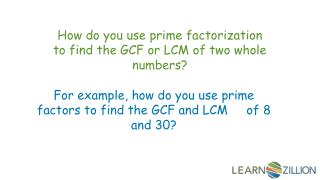DownloadDownload PresentationHow do you use prime factorization to find the GCF or LCM of two whole numbers?

# How do you use prime factorization to find the GCF or LCM of two whole numbers?

Download Presentation## How do you use prime factorization to find the GCF or LCM of two whole numbers?

- - - - - - - - - - - - - - - - - - - - - - - - - - - E N D - - - - - - - - - - - - - - - - - - - - - - - - - - -
##### Presentation Transcript

1. How do you use prime factorization to find the GCF or LCM of two whole numbers? For example, how do you use prime factors to find the GCF and LCM of 8 and 30?

2. In this lesson you will learn how to find the GCF and LCM of two whole numbers by using their prime factors.

3. Prime numbers have only two factors, one and themselves. 7 is a prime number. 7 x 1 = 7 The first few prime numbers are 2, 3, 5, 7, 11, 13, 17… Composite numbers have more than two factors. 10 is a composite number. 1 x 10 = 10 2 x 5 = 10

4. The first few prime numbers are 2, 3, 5, 7, 11, 13, 17… Prime factorization is finding a string of prime numbers that when multiplied result in your original number. factor tree 40 2 x 20 40 = 2 x 2 x 2 x 5 2 x 10 All composite numbers can be written as a string of prime factors. 2 x 5

5. Confusing factors with multiples Find the factors of 25. 1, 5, 25 25, 50, 75…

6. Remembering to find only prime factors for a number when using prime factorization. The prime factors of 20 are 2 x 10.

7. How do you use prime factorization to find the GCF and LCM of 8 and 30? factor tree = 2 x 2 x 2 8 = 2 x 3 x 5 30 2 x 4 2 x 15 2 x 2 3 x 5

8. Venn Diagram 8 GCF = 2 x 2 x 2 30 = 2 x 3 x 5 The GCF of 8 and 30 is 2. The LCM of 8 and 30 is 120. 2 2 2 3 5 LCM 2 x 2 x 2 x 3 x 5 = 120

9. What is the GCF and LCM of 18 and 27? = 3 x 3 x 3 27 = 2 x 3 x 3 18 2 x 9 3 x 9 3 x 3 3 x 3

10. 18 = 2 x 3 x 3 Venn Diagram 3 x 3 = 9 GCF 27 = 3 x 3 x 3 The GCF of 18 and 27 is 9. The LCM of 8 and 30 is 54. 2 3 3 3 LCM 2 x 3 x 3 x 3 = 54

11. In this lesson you have learned how to find the GCF and LCM of two whole numbers by using their prime factors.